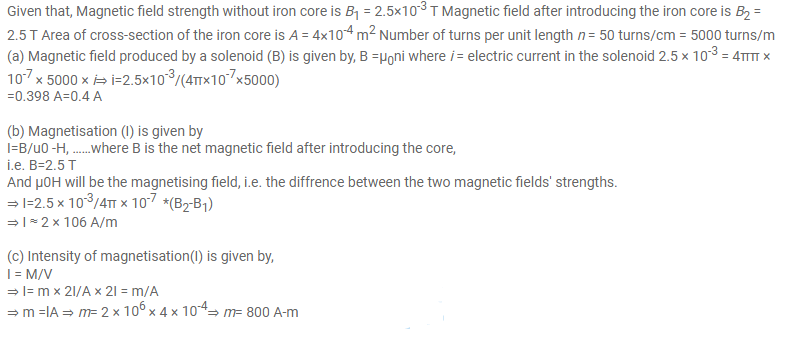# The magnetic field inside a long solenoid of

Question:

The magnetic field inside a long solenoid of 50 turns $\mathrm{cm}^{-1}$ is increased from $2.5 \times 10^{-3} \mathrm{~T}$ to $2.5 \mathrm{~T}$ when an iron core of crosssectional area $4 \mathrm{~cm}^{2}$ is inserted into it. Find

(a) the current in the solenoid

(b) the magnetisation / of the core and

(c) the pole strength developed in the core.

Solution: